Various plots of predictive errors y - yrep. See the Details and Plot Descriptions sections, below.

ppc_error_hist(y, yrep, ..., binwidth = NULL, breaks = NULL, freq = TRUE)

ppc_error_hist_grouped(
y,
yrep,
group,
...,
binwidth = NULL,
breaks = NULL,
freq = TRUE
)

ppc_error_scatter(y, yrep, ..., size = 2.5, alpha = 0.8)

ppc_error_scatter_avg(y, yrep, ..., size = 2.5, alpha = 0.8)

ppc_error_scatter_avg_vs_x(y, yrep, x, ..., size = 2.5, alpha = 0.8)

ppc_error_binned(y, yrep, ..., bins = NULL, size = 1, alpha = 0.25)

## Arguments

y A vector of observations. See Details. An $$S$$ by $$N$$ matrix of draws from the posterior predictive distribution, where $$S$$ is the size of the posterior sample (or subset of the posterior sample used to generate yrep) and $$N$$ is the number of observations (the length of y). The columns of yrep should be in the same order as the data points in y for the plots to make sense. See Details for additional instructions. Currently unused. Passed to ggplot2::geom_histogram() to override the default binwidth. Passed to ggplot2::geom_histogram() as an alternative to binwidth. For histograms, freq=TRUE (the default) puts count on the y-axis. Setting freq=FALSE puts density on the y-axis. (For many plots the y-axis text is off by default. To view the count or density labels on the y-axis see the yaxis_text() convenience function.) A grouping variable (a vector or factor) the same length as y. Each value in group is interpreted as the group level pertaining to the corresponding value of y. For scatterplots, arguments passed to ggplot2::geom_point() to control the appearance of the points. For the binned error plot, arguments controlling the size of the outline and opacity of the shaded region indicating the 2-SE bounds. A numeric vector the same length as y to use as the x-axis variable. For ppc_error_binned(), the number of bins to use (approximately).

## Value

A ggplot object that can be further customized using the ggplot2 package.

## Details

All of these functions (aside from the *_scatter_avg functions) compute and plot predictive errors for each row of the matrix yrep, so it is usually a good idea for yrep to contain only a small number of draws (rows). See Examples, below.

For binomial and Bernoulli data the ppc_error_binned() function can be used to generate binned error plots. Bernoulli data can be input as a vector of 0s and 1s, whereas for binomial data y and yrep should contain "success" proportions (not counts). See the Examples section, below.

## Plot descriptions

ppc_error_hist()

A separate histogram is plotted for the predictive errors computed from y and each dataset (row) in yrep. For this plot yrep should have only a small number of rows.

ppc_error_hist_grouped()

Like ppc_error_hist(), except errors are computed within levels of a grouping variable. The number of histograms is therefore equal to the product of the number of rows in yrep and the number of groups (unique values of group).

ppc_error_scatter()

A separate scatterplot is displayed for y vs. the predictive errors computed from y and each dataset (row) in yrep. For this plot yrep should have only a small number of rows.

ppc_error_scatter_avg()

A single scatterplot of y vs. the average of the errors computed from y and each dataset (row) in yrep. For each individual data point y[n] the average error is the average of the errors for y[n] computed over the the draws from the posterior predictive distribution.

ppc_error_scatter_avg_vs_x()

Same as ppc_error_scatter_avg(), except the average is plotted on the $$y$$-axis and a a predictor variable x is plotted on the $$x$$-axis.

ppc_error_binned()

Intended for use with binomial data. A separate binned error plot (similar to arm::binnedplot()) is generated for each dataset (row) in yrep. For this plot y and yrep should contain proportions rather than counts, and yrep should have only a small number of rows.

Gelman, A., Carlin, J. B., Stern, H. S., Dunson, D. B., Vehtari, A., and Rubin, D. B. (2013). Bayesian Data Analysis. Chapman & Hall/CRC Press, London, third edition. (Ch. 6)

Other PPCs: PPC-censoring, PPC-discrete, PPC-distributions, PPC-intervals, PPC-loo, PPC-overview, PPC-scatterplots, PPC-test-statistics

## Examples

y <- example_y_data()
yrep <- example_yrep_draws()
ppc_error_hist(y, yrep[1:3, ])#> stat_bin() using bins = 30. Pick better value with binwidth.# errors within groups
group <- example_group_data()
(p1 <- ppc_error_hist_grouped(y, yrep[1:3, ], group))#> stat_bin() using bins = 30. Pick better value with binwidth.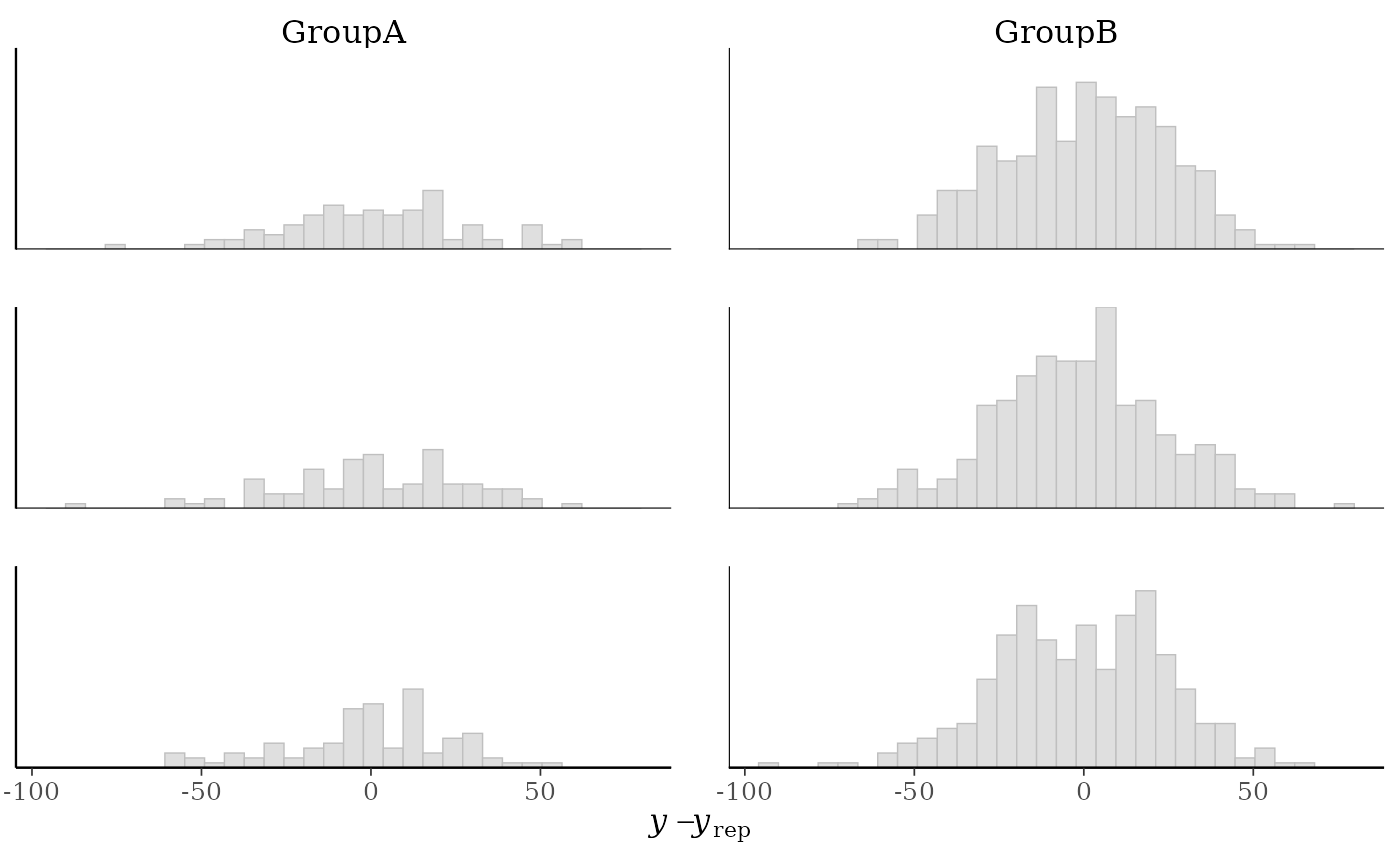p1 + yaxis_text() # defaults to showing counts on y-axis#> stat_bin() using bins = 30. Pick better value with binwidth.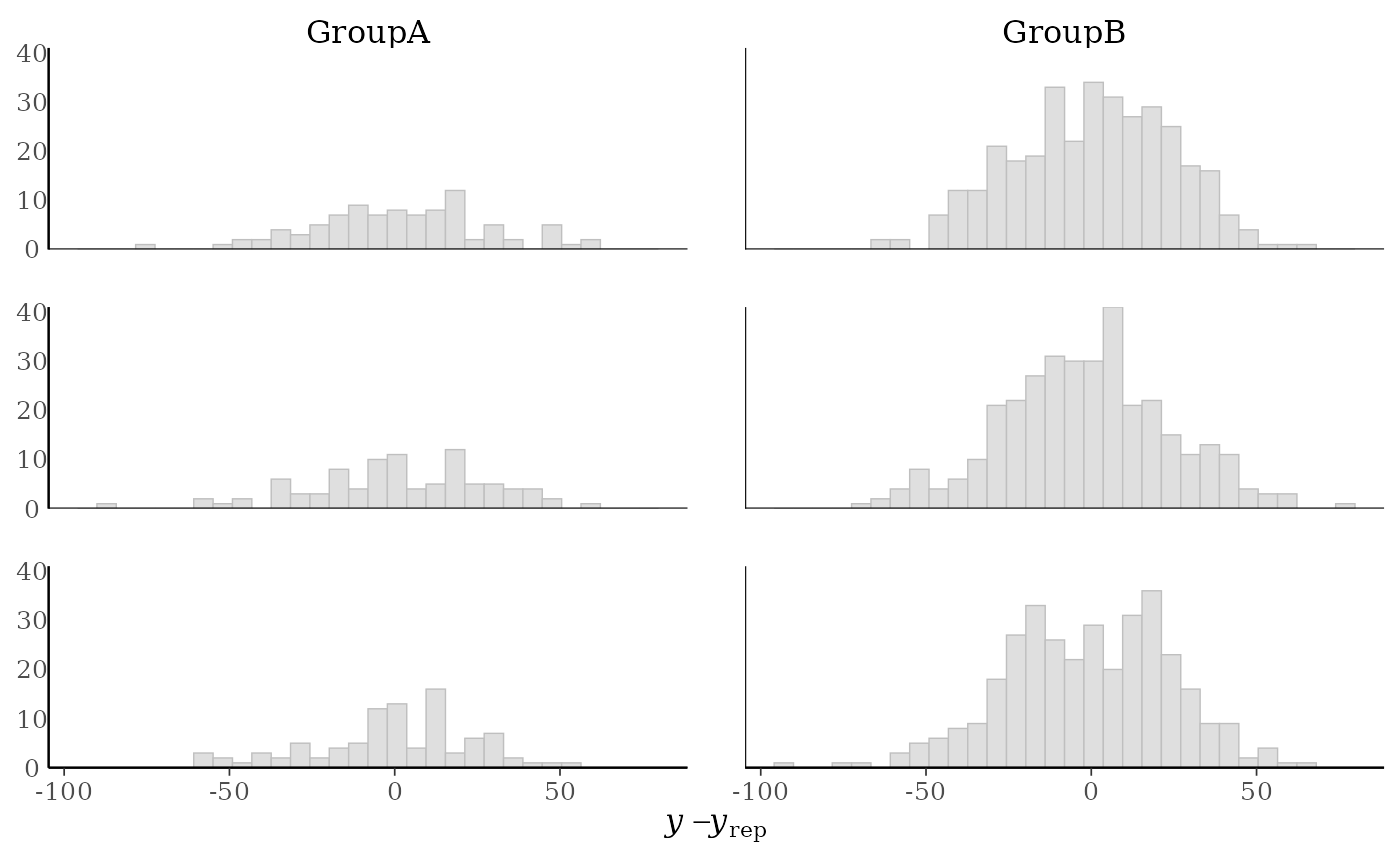# \donttest{
table(group) # more obs in GroupB, can set freq=FALSE to show density on y-axis#> group
#> GroupA GroupB
#>     93    341 (p2 <- ppc_error_hist_grouped(y, yrep[1:3, ], group, freq = FALSE))#> stat_bin() using bins = 30. Pick better value with binwidth.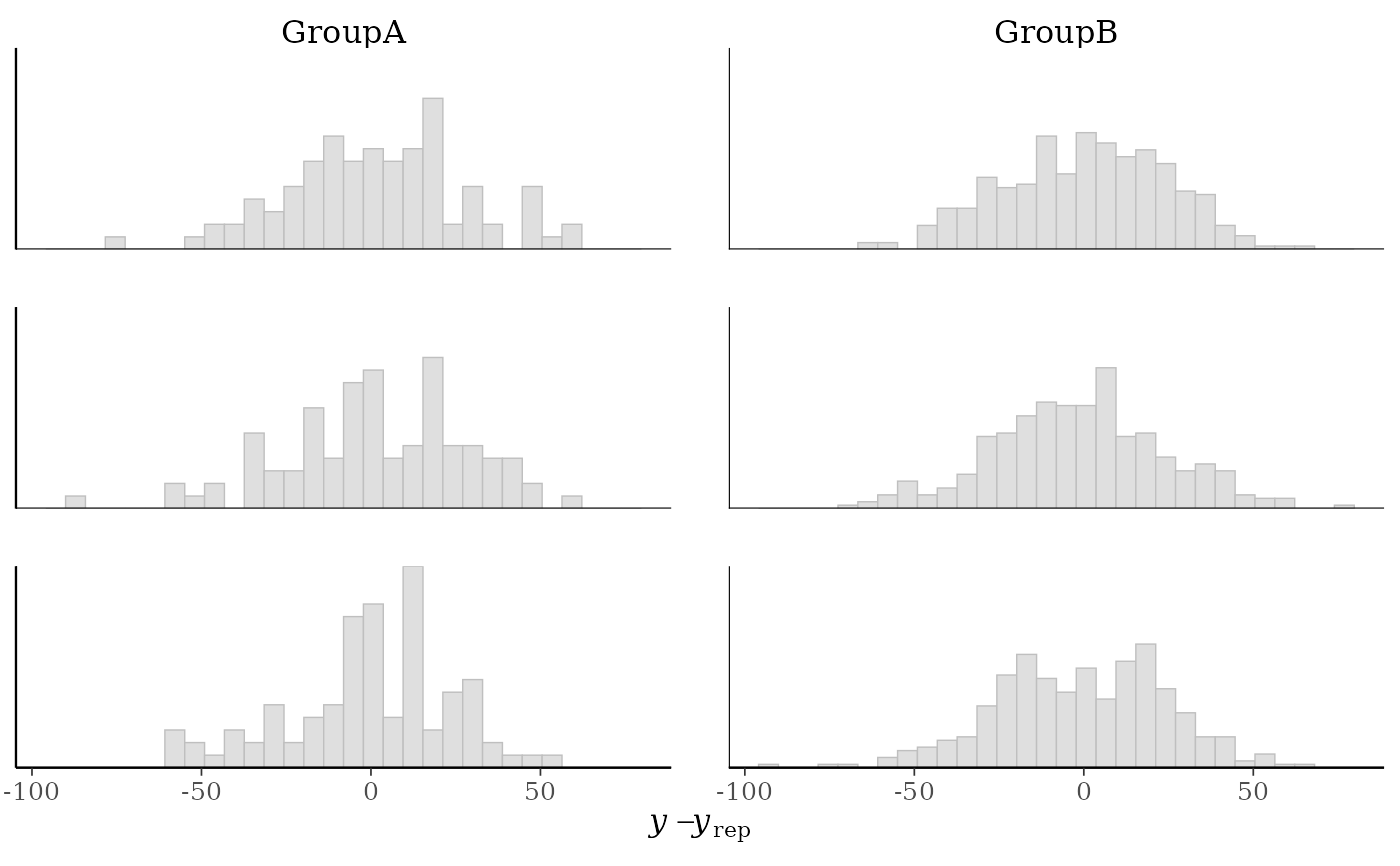p2 + yaxis_text()#> stat_bin() using bins = 30. Pick better value with binwidth.# }

# scatterplots
ppc_error_scatter(y, yrep[10:14, ])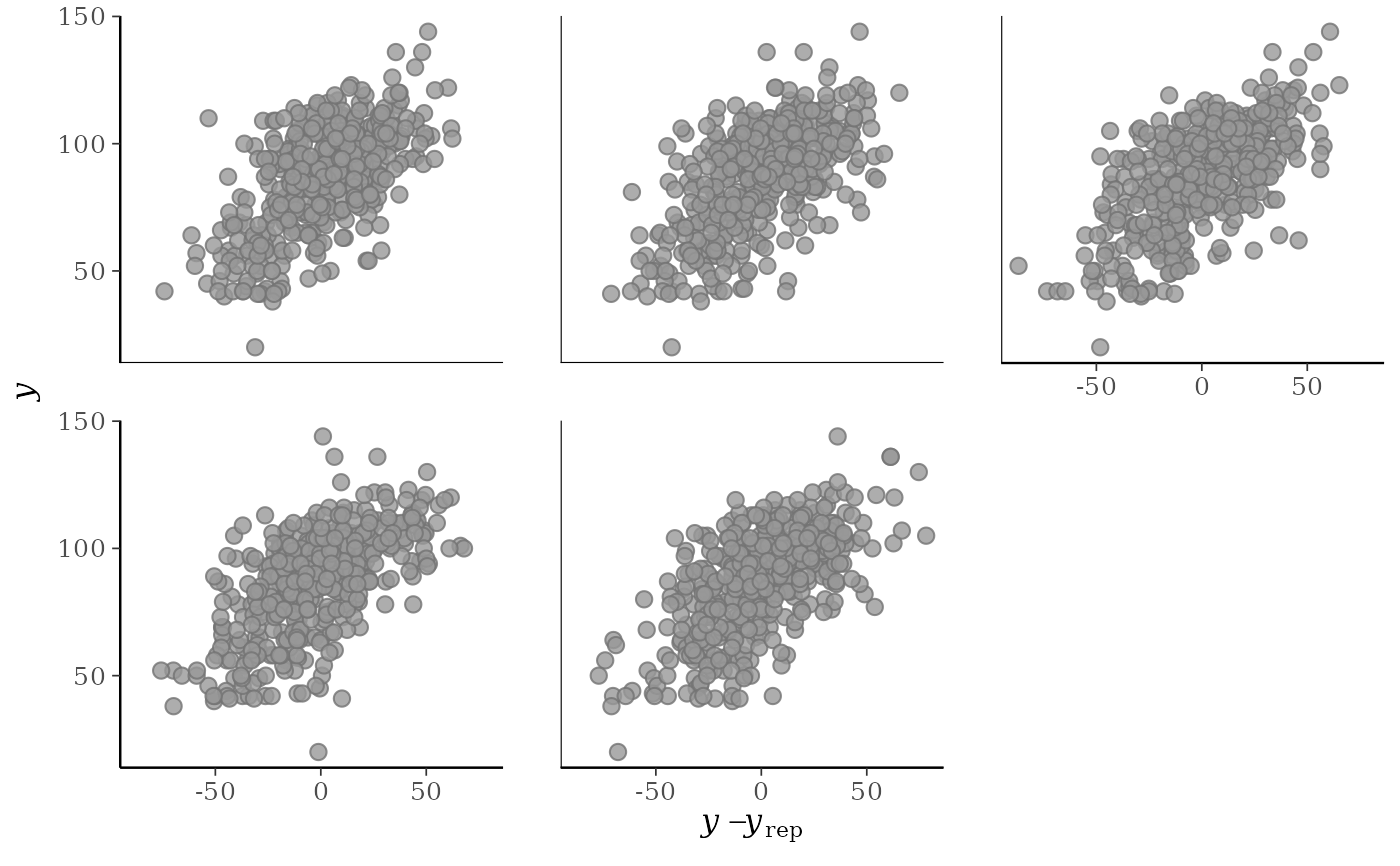ppc_error_scatter_avg(y, yrep)x <- example_x_data()
ppc_error_scatter_avg_vs_x(y, yrep, x)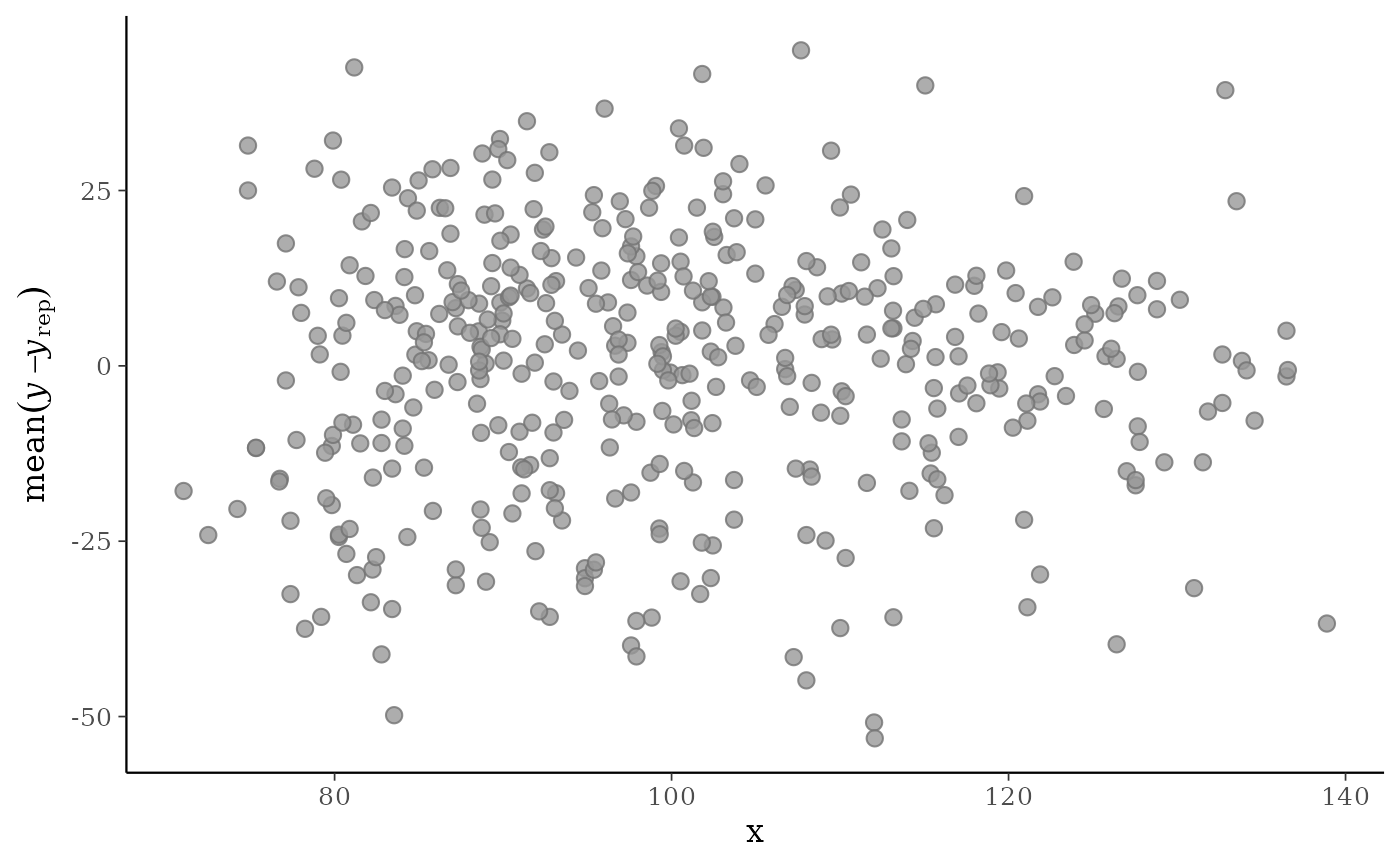# ppc_error_binned with binomial model from rstanarm
# \dontrun{
suppressPackageStartupMessages(library(rstanarm))
suppressWarnings(example("example_model", package = "rstanarm"))#>
#> exmpl_> example_model <-
#> exmpl_+   stan_glmer(cbind(incidence, size - incidence) ~ size + period + (1|herd),
#> exmpl_+              data = lme4::cbpp, family = binomial, QR = TRUE,
#> exmpl_+              # this next line is only to keep the example small in size!
#> exmpl_+              chains = 2, cores = 1, seed = 12345, iter = 1000, refresh = 0)
#>
#> exmpl_> example_model
#> stan_glmer
#>  family:       binomial [logit]
#>  formula:      cbind(incidence, size - incidence) ~ size + period + (1 | herd)
#>  observations: 56
#> ------
#> (Intercept) -1.5    0.6
#> size         0.0    0.0
#> period2     -1.0    0.3
#> period3     -1.1    0.4
#> period4     -1.6    0.5
#>
#> Error terms:
#>  Groups Name        Std.Dev.
#>  herd   (Intercept) 0.79
#> Num. levels: herd 15
#>
#> ------
#> * For help interpreting the printed output see ?print.stanreg
#> * For info on the priors used see ?prior_summary.stanregformula(example_model)#> cbind(incidence, size - incidence) ~ size + period + (1 | herd)
# get observed proportion of "successes"
y <- example_model\$y  # matrix of "success" and "failure" counts
trials <- rowSums(y)
y_prop <- y[, 1] / trials  # proportions

# get predicted success proportions
yrep <- posterior_predict(example_model)
yrep_prop <- sweep(yrep, 2, trials, "/")

ppc_error_binned(y_prop, yrep_prop[1:6, ])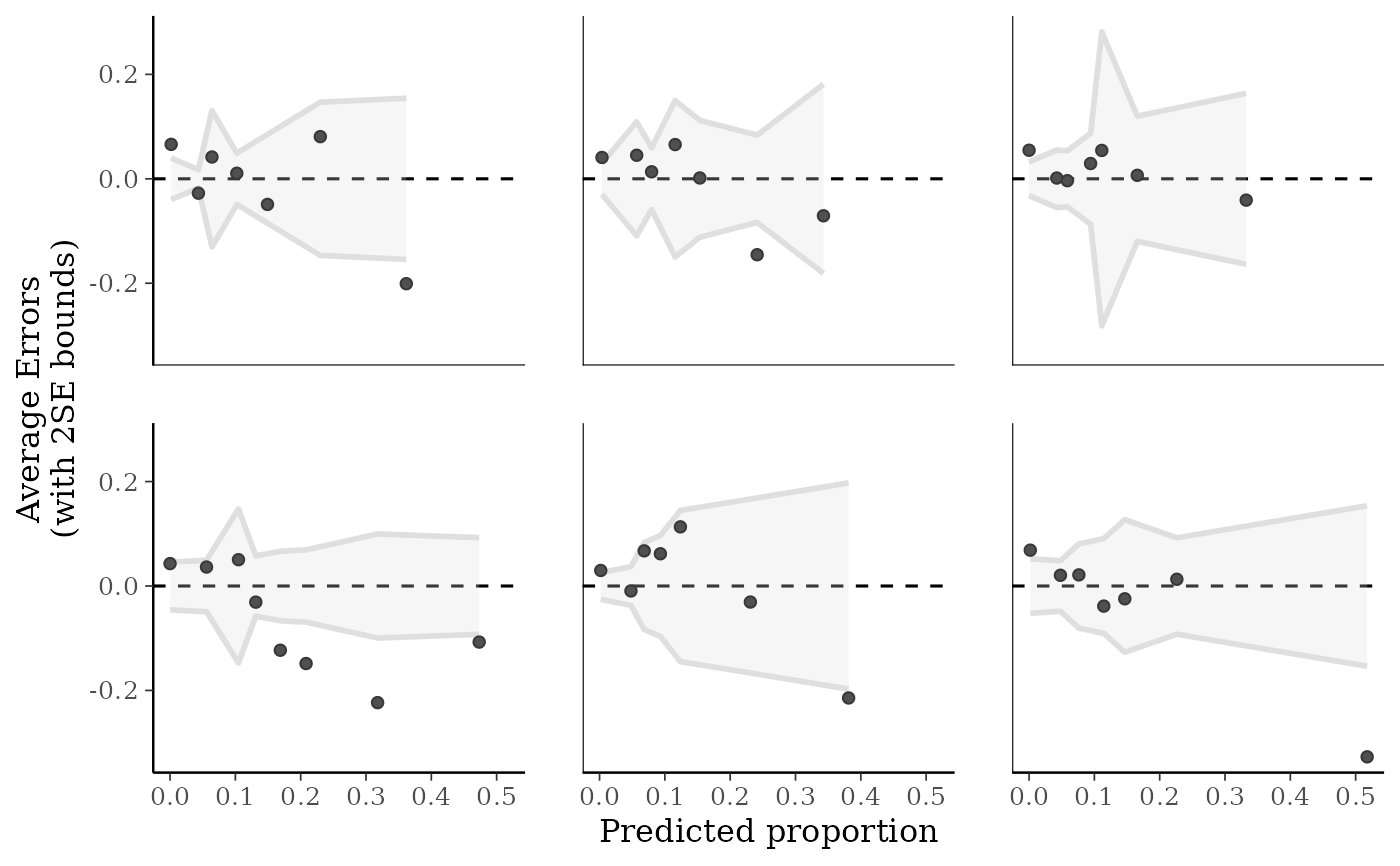# }